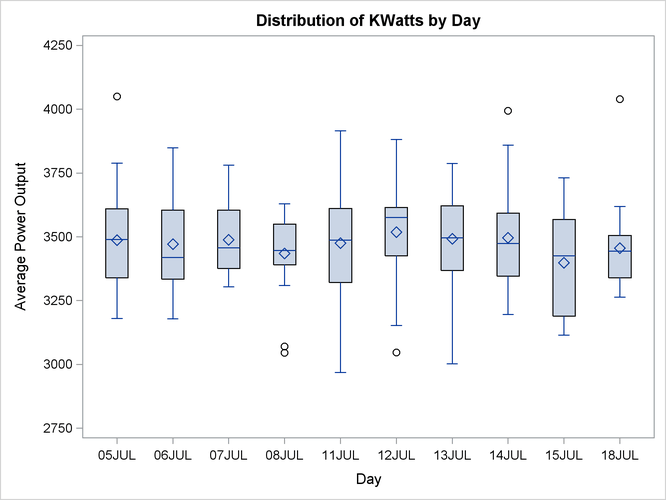### Saving Summary Data with Outliers

In a schematic box plot, outlier values within a group are plotted as separate points beyond the whiskers of the box-and-whiskers plot. See the section Styles of Box Plots and the description of the BOXSTYLE= option for a complete description of schematic box plots.

The following statements use the BOXSTYLE= option to produce a schematic box plot of the data from the `Turbine` data set. The OUTBOX= option creates a summary data set named `OilSchematic`. The ODS GRAPHICS ON statement specified before the PROC BOXPLOT statement enables ODS Graphics, so the box plot is created using ODS Graphics instead of traditional graphics.

```title 'Schematic Box Plot for Power Output';
ods graphics on;
proc boxplot data=Turbine;
plot KWatts*Day / boxstyle = schematic
outbox   = OilSchematic;
run;
```

The schematic box plot is shown in Figure 26.4. Note the outliers plotted for several of the groups.

Figure 26.4: Schematic Box Plot of Power OutputWhereas the `Oilsum` data set from the section Creating Box Plots from Summary Data contains a variable for each summary statistic and one observation per group, the OUTBOX= data set `OilSchematic` contains one observation for each summary statistic in each group. The `_TYPE_` variable identifies the statistic and the `_VALUE_` variable contains its value. In addition, the `OilSchematic` data set contains an observation recording each outlier value for each group. Figure 26.5 shows a partial listing of the `OilSchematic` data set.

Figure 26.5: The Summary Data Set `OilSchematic`

 Schematic Box Plot for Power Output

Day _VAR_ _TYPE_ _VALUE_
05JUL KWatts N 20.00
05JUL KWatts MIN 3180.00
05JUL KWatts Q1 3340.00
05JUL KWatts MEAN 3487.40
05JUL KWatts MEDIAN 3490.00
05JUL KWatts Q3 3610.00
05JUL KWatts MAX 4050.00
05JUL KWatts STDDEV 220.26
05JUL KWatts HIWHISKR 3789.00
05JUL KWatts HIGH 4050.00
06JUL KWatts N 20.00
06JUL KWatts MIN 3179.00
06JUL KWatts Q1 3333.50
06JUL KWatts MEAN 3471.65
06JUL KWatts MEDIAN 3419.50
06JUL KWatts Q3 3605.00
06JUL KWatts MAX 3849.00
06JUL KWatts STDDEV 210.43
07JUL KWatts N 20.00
07JUL KWatts MIN 3304.00
07JUL KWatts Q1 3376.00
07JUL KWatts MEAN 3488.30
07JUL KWatts MEDIAN 3456.50
07JUL KWatts Q3 3604.50
07JUL KWatts MAX 3781.00
07JUL KWatts STDDEV 147.02
08JUL KWatts N 20.00
08JUL KWatts MIN 3045.00
08JUL KWatts Q1 3390.50
08JUL KWatts MEAN 3434.20
08JUL KWatts MEDIAN 3447.00
08JUL KWatts Q3 3550.00
08JUL KWatts MAX 3629.00
08JUL KWatts STDDEV 157.64
08JUL KWatts LOWHISKR 3309.00
08JUL KWatts LOW 3070.00
08JUL KWatts LOW 3045.00
11JUL KWatts N 20.00
11JUL KWatts MIN 2968.00
11JUL KWatts Q1 3321.00

Observations with the `_TYPE_` variable values HIGH and LOW contain outlier values. If you want to use a summary data set to re-create a schematic box plot, you must create an OUTBOX= data set in order to save the outlier data.